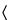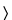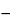Ensulizole
(en sul' i zole).

C13H10N2O3S 274.30
1H-Benzimidazole-5-sulfonic acid-2-phenyl-;
2-Phenylbenzimidazole-5-sulfonic acid[27503-81-7].
DEFINITION
Ensulizole contains NLT 98.0% and NMT 102.0% of C13H10N2O3S, calculated on the dried basis.
IDENTIFICATION
Sample solution:  5 µg/mL
[Note—Transfer 100 mg of Ensulizole to a 100-mL volumetric flask, dissolve in 4 mL of 1 N sodium hydroxide, and dilute with water to volume. Transfer 0.5 mL of the solution to a 100-mL volumetric flask, and dilute with water to volume. ]
Acceptance criteria:  The absorptivities, calculated on the dried basis, at the wavelength of maximum absorbance at about 302 nm, do not differ by more than 3.0%.
ASSAY
•  Procedure
Sample solution:  48 mg/mL of Ensulizole in 0.5 N sodium hydroxide
Titrant:  0.5 N hydrochloric acid VS
Analysis:  To the Sample solution, add phenolphthalein TS, and titrate the excess with Titrant. Perform a blank determination, and make any necessary corrections (see Titrimetry541). Each mL of 0.5 N sodium hydroxide is equivalent to 137.15 mg of C13H10N2O3S.
Calculate the percentage of C13H10N2O3S in the portion of Ensulizole taken:
Result = [(N × (VBVA))/(W × (1LOD))] × Mr1 × 100
 N = = actual normality of the Titrant VB = = volume of Titrant used for the blank (mL) VA = = volume of Titrant used for the Sample solution (mL) W = = weight of Ensulizole taken for the Sample solution (mg) LOD = = percentage of loss on drying expressed as a decimal fraction, as determined in the test for Loss on Drying Mr1 = = molecular weight of ensulizole (274.30)
Acceptance criteria:  98.0%–102.0% on the dried basis
SPECIFIC TESTS
Dry a sample at 105for 4 h: it loses NMT 2.0% of its weight.